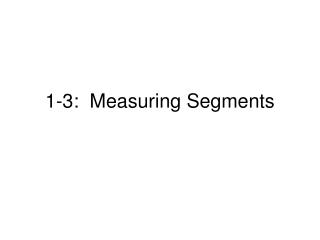DownloadDownload Presentation1-3: Measuring Segments

# 1-3: Measuring Segments

Télécharger la présentation## 1-3: Measuring Segments

- - - - - - - - - - - - - - - - - - - - - - - - - - - E N D - - - - - - - - - - - - - - - - - - - - - - - - - - -
##### Presentation Transcript

1. 1-3: Measuring Segments

2. Ruler Postulate Ruler Postulate: Every point on a line can be paired with a real number. The real number that corresponds to a point is called the coordinate of the point.

3. Distance • The Ruler Postulate allows you to measure lengths of segments using a given unit and to find distances between points on a number line. • The distancebetween points A and B is the absolute value of the difference of their coordinates. AB = |a – b| *Note: AB represents the length of AB.

4. Measuring Segment Lengths • What is ST? • What is UV? • What is SV?

5. Segment Addition Postulate If three points A, B, and C are collinear and B is between A and C, then AB + BC = AC.

6. Using the Segment Addition Postulate • If EG = 59, what are EF and FG?

7. Congruence • When numerical expressions have the same value, you say that they are equal (=). • Similarly, if two segments have the same length, then the segments are congruent () *Note: Segments are can be marked to show congruence.

8. Comparing Segment Lengths • Are AC and BD congruent? • Is AB congruent to DE?

9. Midpoints • The midpoint of a segment is a point that divides the segment into two congruent segments. • A point, line, ray, etc. that intersects a segment at its midpoint is said to bisectthe segment and is called a segment bisector.

10. Using the Midpoint • If Q is the midpoint, what are PQ, QR, and PR?